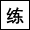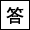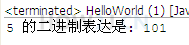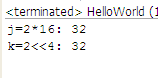操作符系列教材 （三）- Java的逻辑运算符 操作符系列教材 （五）- Java的赋值操作符11分46秒

Integer.toBinaryString()

|

&

^

~

<<
>>>>>## 一个整数的二进制表达public class HelloWorld { public static void main(String[] args) { int i = 5; String b = (Integer.toBinaryString(i)); // 5的二进制的表达101 System.out.println(i+" 的二进制表达是: "+b); } }
```public class HelloWorld {
public static void main(String[] args) {
int i = 5;
String b = (Integer.toBinaryString(i)); // 5的二进制的表达101
System.out.println(i+" 的二进制表达是: "+b);
}
}
```

## 位或

5的二进制是101
6的二进制是110

public class HelloWorld { public static void main(String[] args) { int i =5; int j = 6; System.out.println(Integer.toBinaryString(i)); //5的二进制是101 System.out.println(Integer.toBinaryString(j)); //6的二进制是110 System.out.println(i|j); //所以 5|6 对每一位进行或运算，得到 111->7 } }
```public class HelloWorld {
public static void main(String[] args) {

int i  =5;
int j = 6;

System.out.println(Integer.toBinaryString(i)); //5的二进制是101

System.out.println(Integer.toBinaryString(j)); //6的二进制是110

System.out.println(i|j); //所以 5|6 对每一位进行或运算，得到 111->7

}
}
```

## 位与

5的二进制是101
6的二进制是110

public class HelloWorld { public static void main(String[] args) { int i =5; int j = 6; System.out.println(Integer.toBinaryString(i)); //5的二进制是101 System.out.println(Integer.toBinaryString(j)); //6的二进制是110 System.out.println(i&j); //所以 5&6 对每一位进行与运算，得到 100->4 } }
```public class HelloWorld {
public static void main(String[] args) {

int i  =5;
int j = 6;

System.out.println(Integer.toBinaryString(i)); //5的二进制是101

System.out.println(Integer.toBinaryString(j)); //6的二进制是110

System.out.println(i&j); //所以 5&6 对每一位进行与运算，得到 100->4

}
}
```

## 异或

5的二进制是101
6的二进制是110

public class HelloWorld { public static void main(String[] args) { int i =5; int j = 6; System.out.println(Integer.toBinaryString(i)); //5的二进制是 101 System.out.println(Integer.toBinaryString(j)); //6的二进制是110 System.out.println(i^j); //所以 5^6 对每一位进行或运算，得到 011->3 System.out.println(i^0); System.out.println(i^i); } }
```public class HelloWorld {
public static void main(String[] args) {
int i  =5;
int j = 6;
System.out.println(Integer.toBinaryString(i)); //5的二进制是 101
System.out.println(Integer.toBinaryString(j)); //6的二进制是110
System.out.println(i^j); //所以 5^6 对每一位进行或运算，得到 011->3

System.out.println(i^0);
System.out.println(i^i);
}
}
```

## 取非

5 的二进制是 00000101

public class HelloWorld { public static void main(String[] args) { byte i =5; System.out.println(Integer.toBinaryString(i)); //5的二进制是00000101,所以取非即为11111010,即为-6 System.out.println(~i); } }
```public class HelloWorld {
public static void main(String[] args) {
byte i  =5;

System.out.println(Integer.toBinaryString(i)); //5的二进制是00000101,所以取非即为11111010,即为-6

System.out.println(~i);

}

}
```

## 左移 右移

public class HelloWorld { public static void main(String[] args) { byte i =6; //6的二进制是110 System.out.println(Integer.toBinaryString(i)); //6向左移1位后，变成1100，对应的10进制是12 System.out.println(i<<1); //6向右移1位后，变成11，对应的10进制是3 System.out.println(i>>1); } }
```public class HelloWorld {
public static void main(String[] args) {
byte i  =6;

//6的二进制是110
System.out.println(Integer.toBinaryString(i));
//6向左移1位后，变成1100，对应的10进制是12
System.out.println(i<<1);
//6向右移1位后，变成11，对应的10进制是3
System.out.println(i>>1);
}

}
```

## 练习-快速计算2x16

姿势不对,事倍功半! 点击查看做练习的正确姿势

## 答案-快速计算2x161分54秒 本视频采用html5方式播放，如无法正常播放，请将浏览器升级至最新版本，推荐火狐，chrome，360浏览器。 如果装有迅雷，播放视频呈现直接下载状态，请调整 迅雷系统设置-基本设置-启动-监视全部浏览器 (去掉这个选项)。 chrome 的 视频下载插件会影响播放，如 IDM 等，请关闭或者切换其他浏览器public class HelloWorld { public static void main(String[] args) { int i = 2; int j = 2*16; //使用乘法 System.out.println("j=2*16:\t"+j); int k = 2<<4; System.out.println("k=2<<4:\t"+k); } }
```public class HelloWorld {
public static void main(String[] args) {

int i = 2;
int j = 2*16; //使用乘法
System.out.println("j=2*16:\t"+j);
int k = 2<<4;
System.out.println("k=2<<4:\t"+k);
}
}
```

## 带符号右移与无符号右移

public class HelloWorld { public static void main(String[] args) { int i =-10; //-10的二进制是11111111111111111111111111110110 //第一位是1，即符号位，代表这是一个负数 System.out.println(Integer.toBinaryString(i)); //对于正数， 带符号右移 >> 会把所有的位右移，并在最前面补0 //对于负数， 带符号右移 >> 会把所有的位右移，并在最前面补1 //-10带符号右移1位，移动后前面补齐1 //得到11111111111111111111111111111011 //因为第一位是1，所以依然是一个负数，对应的十进制是-5 int j = i>>1; System.out.println(Integer.toBinaryString(j)); System.out.println(j); //-10无符号向右移1位，符号位也会向右移，第一位就变成了0 //得到01111111111111111111111111111011，对应的十进制是2147483643 int k = i>>>1; System.out.println(Integer.toBinaryString(k)); System.out.println(k); } }

## 练习-位操作符

姿势不对,事倍功半! 点击查看做练习的正确姿势

int i = 3; // 二进制是11
int j = 2; // 二进制是10
int c = ((i | j) ^ (i & j)) << 2 >>> 1;

## 答案-位操作符1分7秒 本视频采用html5方式播放，如无法正常播放，请将浏览器升级至最新版本，推荐火狐，chrome，360浏览器。 如果装有迅雷，播放视频呈现直接下载状态，请调整 迅雷系统设置-基本设置-启动-监视全部浏览器 (去掉这个选项)。 chrome 的 视频下载插件会影响播放，如 IDM 等，请关闭或者切换其他浏览器

public class HelloWorld { public static void main(String[] args) { int i = 3; // 二进制是11 int j = 2; // 二进制是10 int c = ((i | j) ^ (i & j)) << 2 >>> 1; // 11 // 10 // 01 // 0100 // 010 //010=2 } }
```public class HelloWorld {
public static void main(String[] args) {
int i = 3; // 二进制是11
int j = 2; // 二进制是10
int c = ((i | j) ^ (i & j)) << 2 >>> 1;
//		    11
//					  10
//			     01
//							  0100
//									010
//010=2
}
}
```

 操作符系列教材 （三）- Java的逻辑运算符 操作符系列教材 （五）- Java的赋值操作符

HOW2J公众号，关注后实时获知最新的教程和优惠活动，谢谢。2021-09-27 int c = ((i | j) ^ (i & j)) << 2 >>> 1;是怎么算的？
 zxl271211 关于 JAVA 基础-操作符-位操作符 的提问 为什么是 >>> 1，而不是>> 1？ `int c = ((i | j) ^ (i & j)) << 2 >>> 1`

2 个答案

```1.((11)^(10))<<2>>>1;
2.(01)<<2>>>1;
3.(100)>>>1;
4.10 (十进制表达就是2)```

Lr_Eliauk

2021-08-01 为什么转二进制不能转浮点数
 Beic 关于 JAVA 基础-操作符-位操作符 的提问 转二进制为什么只能转整数 不能转小数 ```import java.util.Scanner; public class Zhuan2jinzhi { public static void main(String[] args) { Scanner a = new Scanner(System.in); // double b = a.nextDouble(); int b = a.nextInt(); String c = (Integer.toBinaryString(b)); System.out.println(b+"的二进制为:"+c); } } ```

2 个答案

park

2021-07-30 答案
2021-07-04 示例8在eclipse里面输出的第四个数据没有32位，只有31位，二进制输出有误啊！！！！！
2021-06-08 5 取非为什么为-6